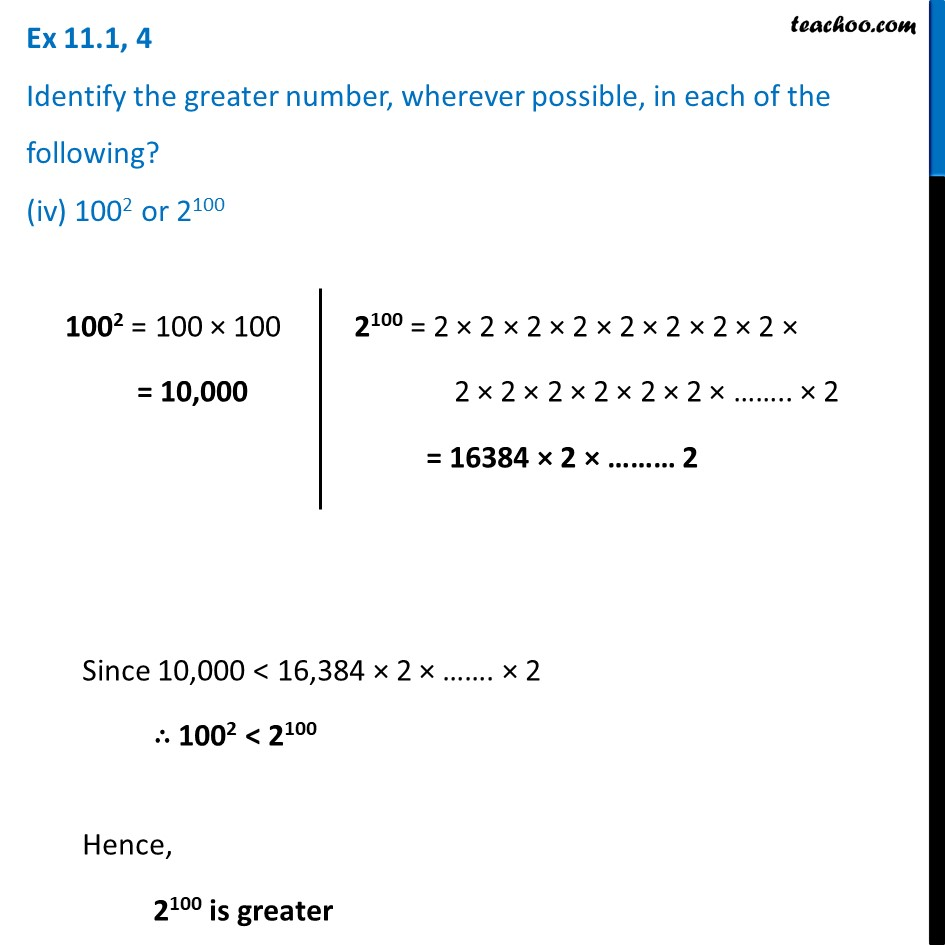Ex 11.1

Chapter 11 Class 7 Exponents and Powers
Serial order wiseLearn in your speed, with individual attention - Teachoo Maths 1-on-1 Class

### Transcript

Ex 11.1, 4 Identify the greater number, wherever possible, in each of the following? (iv) 1002 or 2100 1002 = 100 × 100 = 10,000 = 2 × 2 × 2 × 2 × 2 × 2 × 2 × 2 × 2 × 2 × 2 × 2 × 2 × 2 × …….. × 2 = 16384 × 2 × ……… 2 Since 10,000 < 16,384 × 2 × ……. × 2 ∴ 1002 < 2100 Hence, 2100 is greater